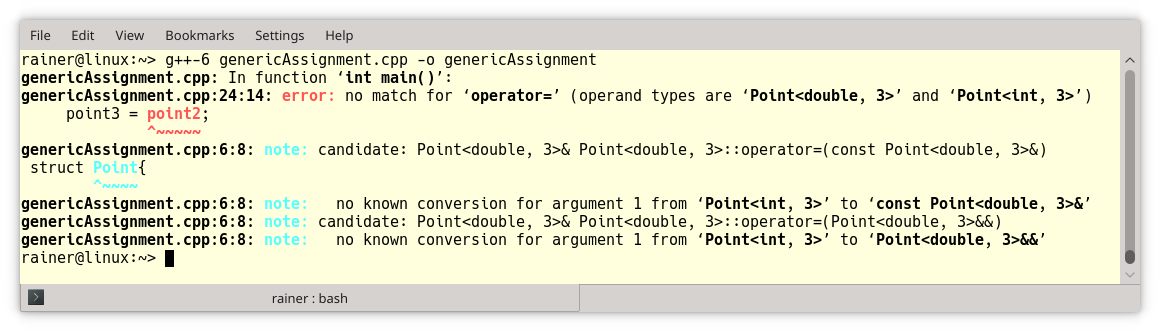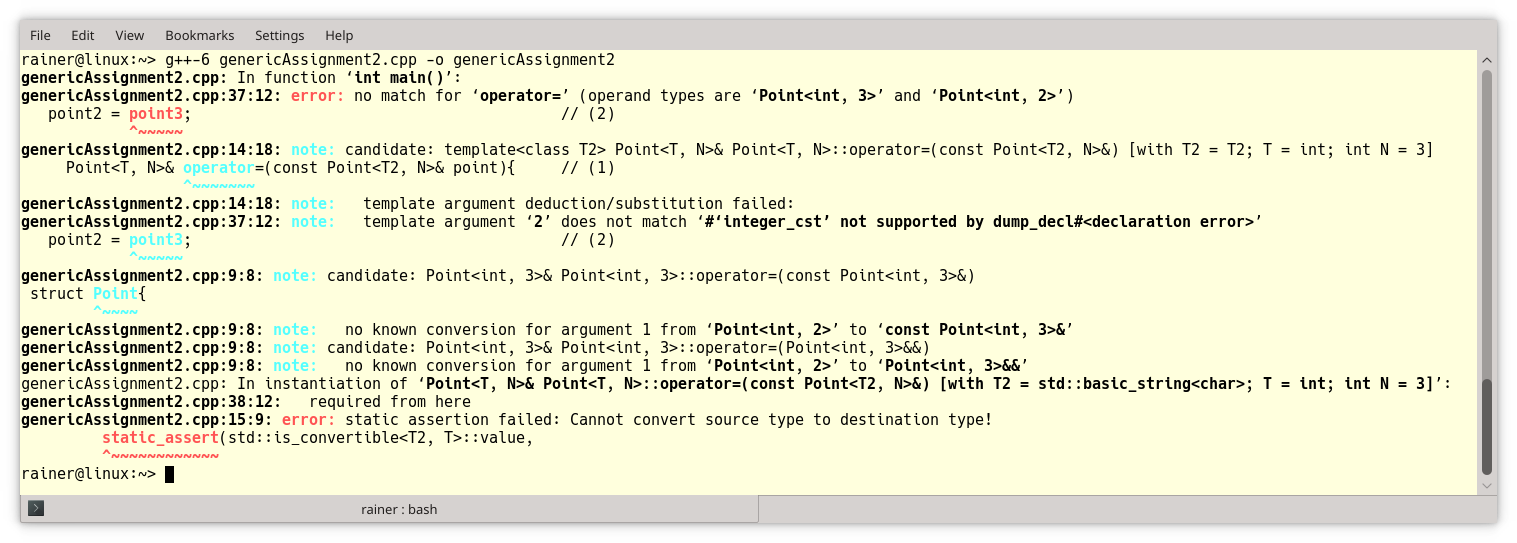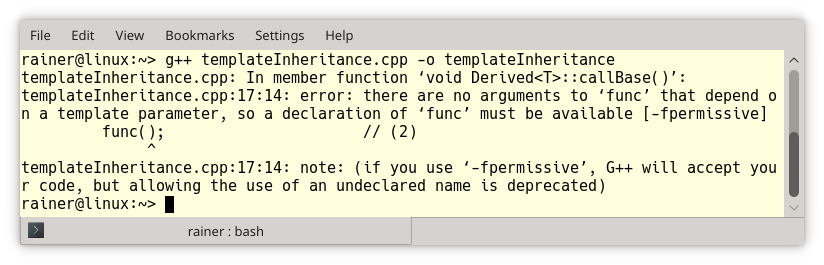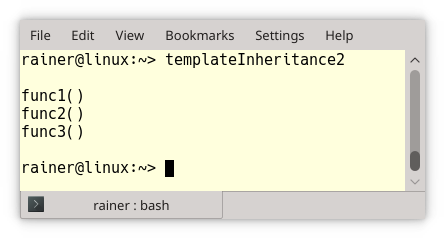# Templates: Misconceptions and Surprises

I often teach the basics of templates. Templates are special. Therefore, I encounter many misconceptions which cause surprises. Here are a few of them.My first misconception is presumably apparent for many but not all C++ developers.

## Templates of Related Types are not Related

First of all, what does the related type mean? My informal term stands for types that can be implicitly converted. Here is the starting point.

```// genericAssignment.cpp

#include <vector>

template <typename T, int N>             // (1)
struct Point{
Point(std::initializer_list<T> initList): coord(initList){}

std::vector<T> coord;
};

int main(){

Point<int, 3> point1{1, 2, 3};
Point<int, 3> point2{4, 5, 6};

point1 = point2;                    // (2)

auto doubleValue = 2.2;
auto intValue = 2;
doubleValue = intValue;             // (3)

Point<double, 3> point3{1.1, 2.2, 3.3};
point3 = point2;                    // (4)

}
```

The class template Point stands for a point in an n-dimensional space. The type of the coordinates and the dimension can be adjusted (line 1). The coordinates are stored in a std::vector<T>. When I create two points with the same coordinate type and dimension, I can assign them.

##Modernes C++ Mentoring

Be part of my mentoring programs:

• "Fundamentals for C++ Professionals" (open)
• "Design Patterns and Architectural Patterns with C++" (open)
• "C++20: Get the Details" (reopens December 2023)
• Do you want to stay informed about my mentoring programs: Subscribe via E-Mail.

Now the misconception begins. You can assign an int to a double (line 3). Therefore, assigning a Point of ints to the point of doubles should be possible. The C++ compiler is quite specific about line 4. Both class templates are not related and cannot be assigned. They are different types.The error message gives the first hint. I need an assignment operator that supports the conversion from Point<int, 3> to Point<double, 3>. The class template now has a generic copy assignment operator.

```// genericAssignment2.cpp

#include <algorithm>
#include <iostream>
#include <string>
#include <vector>

template <typename T, int N>
struct Point{

Point(std::initializer_list<T> initList): coord(initList){}

template <typename T2>
Point<T, N>& operator=(const Point<T2, N>& point){     // (1)
static_assert(std::is_convertible<T2, T>::value,
"Cannot convert source type to destination type!");
coord.clear();
coord.insert(coord.begin(), point.coord.begin(), point.coord.end());
return *this;
}

std::vector<T> coord;

};

int main(){

Point<double, 3> point1{1.1, 2.2, 3.3};
Point<int, 3> point2{1, 2, 3};

Point<int, 2> point3{1, 2};
Point<std::string, 3> point4{"Only", "a", "test"};

point1 = point2;                                        // (3)

// point2 = point3;                                     // (4)
// point2 = point4;                                     // (5)

}
```

Due to line (1), the copy assignment in line (3) works. Let’s have a closer look at the class template Point:

• Point<T, N>& operator=(const Point<T2, N>& point): The assigned to Point is of type Point<T, N> and accepts only the Point, which has the same dimension, but the type could vary: Point<T2, N>.
• static_assert(std::is_convertible<T2, T>::value, “Cannot convert source type to destination type!”): This expression checks with the help of the function std::is_convertible from the type-traits library, if T2 can be converted to T.

When I use lines (4) and (5), the compilation fails:Line (3) gives an error because both points have different dimensions. Line (4) triggers the static_assert in the assignment operator because a std::string is not convertible to an int.

I assume the next misconception has more surprise potential.

## Methods inherited from Class Templates are per se not available

Let’s start simple.

```// inheritance.cpp

#include <iostream>

class Base{
public:
void func(){                    // (1)
std::cout << "func" << std::endl;
}
};

class Derived: public Base{
public:
void callBase(){
func();                      // (2)
}
};

int main(){

std::cout << std::endl;

Derived derived;
derived.callBase();

std::cout << std::endl;

}
```

I implemented a class, Base, and Derived. Derived is public derived from Base and can, therefore, be used in its method callBase (line 2), the method func from class Base. Okay, I have nothing to add to the output of the program.Making Base a class template changes the behavior.

```// templateInheritance.cpp

#include <iostream>

template <typename T>
class Base{
public:
void func(){                    // (1)
std::cout << "func" << std::endl;
}
};

template <typename T>
class Derived: public Base<T>{
public:
void callBase(){
func();                      // (2)
}
};

int main(){

std::cout << std::endl;

Derived<int> derived;
derived.callBase();

std::cout << std::endl;

}
```

I assume the compiler error may surprise you.The line “there are no arguments to ‘func’ that depend on a template parameter, so a declaration of ‘func’ must be available” from the error message gives the first hint. func is a so-called non-dependent name because its name does not depend on the template parameter T. The consequence is that the compiler does not look in the from T dependent base class Base<T>, and there is no name func available outside the class template.

There are three workarounds to extend the name lookup to the dependent base class. The following example uses all three.

```// templateInheritance2.cpp

#include <iostream>

template <typename T>
class Base{
public:
void func1() const {
std::cout << "func1()" << std::endl;
}
void func2() const {
std::cout << "func2()" << std::endl;
}
void func3() const {
std::cout << "func3()" << std::endl;
}
};

template <typename T>
class Derived: public Base<T>{
public:
using Base<T>::func2;              // (2)
void callAllBaseFunctions(){

this->func1();                   // (1)
func2();                         // (2)
Base<T>::func3();                // (3)

}
};

int main(){

std::cout << std::endl;

Derived<int> derived;
derived.callAllBaseFunctions();

std::cout << std::endl;

}
```

• Make the name dependent: The call this->func1 in line 1 is dependent because this is implicit dependent. The name lookup will consider, in this case, all base classes.
• Introduce the name into the current scope: The expression using Base<T>::func2 (line 2) introduces func2 into the current scope.
• Call the name fully qualified: Calling func3 fully qualified (line 3) will break a virtual dispatch and may cause new surprises.

In the end, here is the output of the program.## What’s next?

I have more to write about dependent names in my next post. Sometimes you have to disambiguate dependent names with typename or template. If you see this for the first time, you are probably as surprised as me.

Thanks a lot to my Patreon Supporters: Matt Braun, Roman Postanciuc, Tobias Zindl, G Prvulovic, Reinhold Dröge, Abernitzke, Frank Grimm, Sakib, Broeserl, António Pina, Sergey Agafyin, Андрей Бурмистров, Jake, GS, Lawton Shoemake, Jozo Leko, John Breland, Venkat Nandam, Jose Francisco, Douglas Tinkham, Kuchlong Kuchlong, Robert Blanch, Truels Wissneth, Kris Kafka, Mario Luoni, Friedrich Huber, lennonli, Pramod Tikare Muralidhara, Peter Ware, Daniel Hufschläger, Alessandro Pezzato, Bob Perry, Satish Vangipuram, Andi Ireland, Richard Ohnemus, Michael Dunsky, Leo Goodstadt, John Wiederhirn, Yacob Cohen-Arazi, Florian Tischler, Robin Furness, Michael Young, Holger Detering, Bernd Mühlhaus, Matthieu Bolt, Stephen Kelley, Kyle Dean, Tusar Palauri, Dmitry Farberov, Juan Dent, George Liao, Daniel Ceperley, Jon T Hess, Stephen Totten, Wolfgang Fütterer, Matthias Grün, Phillip Diekmann, Ben Atakora, Ann Shatoff, Rob North, and Bhavith C Achar.

Thanks, in particular, to Jon Hess, Lakshman, Christian Wittenhorst, Sherhy Pyton, Dendi Suhubdy, Sudhakar Belagurusamy, Richard Sargeant, Rusty Fleming, John Nebel, Mipko, Alicja Kaminska, Slavko Radman, and David Poole.

 My special thanks to EmbarcaderoMy special thanks to PVS-StudioMy special thanks to Tipi.buildMy special thanks to Take Up Code## Seminars

I’m happy to give online seminars or face-to-face seminars worldwide. Please call me if you have any questions.

### Standard Seminars (English/German)

Here is a compilation of my standard seminars. These seminars are only meant to give you a first orientation.

• C++ – The Core Language
• C++ – The Standard Library
• C++ – Compact
• C++11 and C++14
• Concurrency with Modern C++
• Design Pattern and Architectural Pattern with C++
• Embedded Programming with Modern C++
• Generic Programming (Templates) with C++

#### New

• Clean Code with Modern C++
• C++20

### Contact Me

Modernes C++ Mentoring,0 replies